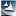# 模拟退火算法求最优解(转载)2 模拟退火算法流程图

1) 冷却参数表初始化：

①初始温度T一般要求T的初始值要充分大，即一开始处于高温状态。

②温度的衰减因子。衰减函数用于控制温度的退火速度，一个常用的函数为：T(n + 1) = K*T(n)，其中K是一个非常接近于1的常数。

③搜索步长因子。

④每个T值的迭代次数L(马可夫链长度)在衰减参数T的衰减函数已选定的前提下，L应选得在控制参数的每一取值上都能恢复准平衡。即每一次随机游走过程，要迭代多少次，才能趋于一个准平衡分布，即一个局部收敛解位置。

⑤初始解状态i。是算法迭代的起点

⑥结束条件S有很多种终止条件的选择，各种不同的条件对算法的性能和解的质量有很大影响，本文只介绍一个常用的终止条件。即上一个最优解与最新的一个最优解的之差小于某个容差，即可停止此次马尔可夫链的迭代。

2) 对k=1，…，L做第3至第5步

3) 产生新解S′的新解产生器。

4) Metropolis准则

(1) Δt′= f(X(i+1)) - f(X(i)) < 0 (即移动后得到更优解)，则总是接受该移动，将新解S′作为当前解S。

(2) Δt= f(X(i+1)) - J(X(i)) > 0 (即移动后的解比当前解要差)，则以一定的概率接受移动，而且这个概率随着时间推移逐渐降低（逐渐降低才能趋向稳定）。

5) 如果满足终止条件则输出当前解作为最优解，结束程序。

6) T逐渐减少，且T->0，然后转第2步。

1) 伪代码：

while(T > T_min)

{

dE = f(Y(i+1)) - f(Y(i));

if(dE>=0)//表达移动后得到更优解，则总是接受移动

Y(i+1) = Y(i); //接受从Y(i)到Y(i+1)的移动

else

{

//函数exp(dE/T)的取值范围是(0,1)，dE/T越大，则exp(dE/T)也越大

if(exp(dE/T) > random(0,1))

Y(i+1)=Y(i);//接受从Y(i)到Y(i+1)的移动

}

T=r * T; //降温退火

i++;

}

2) C#代码

详细代码见Program.cs

https://blog.sciencenet.cn/blog-1813407-893984.html

## 全部精选博文导读

GMT+8, 2023-6-7 22:40